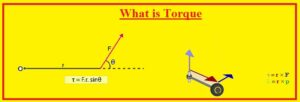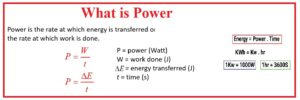Hello, readers welcome to the new post. We will discuss Difference between power and torque. Power discusses the rate of work done and torque is energy that is given when an object rotates about an axis.

In this post, we will discuss different paramerts to learn the basic differences between these two terms. So let’s get started Difference between power and torque.

## Difference between power and torque

What is Power

• Energy is converted and transferred in unit time called power. The unit of power is a watt which is one-joule work done in one second
• It is also called activity performed and scalar quantity.
• Power can be related to other paramerts such as power used for movement of the vehicle on road is multiple of traction forces and velocity.
• The equation of power is
• P= ∆E/ ∆t
• P is power  ∆E is energy variation ∆t change in time
• SI unit of power is watt
• Power is generated through a generator and battery
• There are 2 main types of power electrical and mechanical power
• One horsepower is equal to 746 watts
• here are three types of electrical system power apparent real and reactive power.
• The apparent power is multiple of RMS volts and current and also called idle power
• Active power is called real power used in the circuitry denoted as P. Active power is used in resistive circuits.
• Power used in circuitry reactance is called reactive power. Denoted with Q and unit is MVR### What is Torque• Torque is a rotation correspondent of linear force. It is also named a moment of force, rotation force, and turning effect.
• It denotes the ability of the force to generate variation in the rotational motion of objects.
• Torque is explained as multiple of the magnitude of the force and the perpendicular distance of linear applications of force through rotation of the axis.
• The formula of torque is
• Torque = FOrce x distance
• It is a vector quantity and the unit is NM
• Torque meter used for torque value

That is all about the difference between power and torque. All details has been explained if you have any further query ask in comments. Thanks for reading have a good day see you in next post.